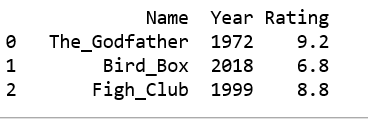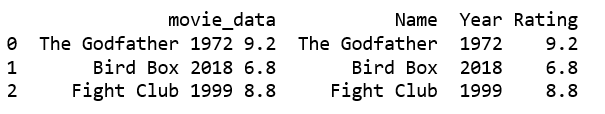# Split a String into columns using regex in pandas DataFrame

Given some mixed data containing multiple values as a string, let’s see how can we divide the strings using regex and make multiple columns in Pandas DataFrame.

Method #1:
In this method we will use `re.search(pattern, string, flags=0)`. Here pattern refers to the pattern that we want to search. It takes in a string with the following values:

• \w matches alphanumeric characters
• \d matches digits, which means 0-9
• \s matches whitespace characters
• \S matches non-whitespace characters
• . matches any character except the new line character \n
• * matches 0 or more instances of a pattern

 `# import the regex library ` `import` `pandas as pd ` `import` `re ` ` `  `# Create a list with all the strings ` `movie_data ``=` `[``"Name: The_Godfather Year: 1972 Rating: 9.2"``, ` `            ``"Name: Bird_Box Year: 2018 Rating: 6.8"``, ` `            ``"Name: Fight_Club Year: 1999 Rating: 8.8"``] ` ` `  `# Create a dictionary with the required columns  ` `# Used later to convert to DataFrame ` `movies ``=` `{``"Name"``:[], ``"Year"``:[], ``"Rating"``:[]} ` ` `  `for` `item ``in` `movie_data: ` `     `  `    ``# For Name field ` `    ``name_field ``=` `re.search(``"Name: .*"``,item) ` `     `  `    ``if` `name_field ``is` `not` `None``: ` `        ``name ``=` `re.search(``'\w*\s\w*'``,name_field.group()) ` `    ``else``: ` `        ``name ``=` `None` `    ``movies[``"Name"``].append(name.group()) ` `     `  `    ``# For Year field ` `    ``year_field ``=` `re.search(``"Year: .*"``,item) ` `    ``if` `year_field ``is` `not` `None``: ` `        ``year ``=` `re.search(``'\s\d\d\d\d'``,year_field.group()) ` `    ``else``: ` `        ``year ``=` `None` `    ``movies[``"Year"``].append(year.group().strip()) ` `     `  `    ``# For rating field ` `    ``rating_field ``=` `re.search(``"Rating: .*"``,item) ` `    ``if` `rating_field ``is` `not` `None``:  ` `        ``rating ``=` `re.search(``'\s\d.\d'``,rating_field.group()) ` `    ``else``:  ` `        ``rating ``-` `None` `    ``movies[``"Rating"``].append(rating.group().strip()) ` ` `  `# Creating DataFrame ` `df ``=` `pd.DataFrame(movies) ` `print``(df) `

Output:Explanation:

• In the code above, we use a for loop to iterate through movie data so we can work with each movie in turn. We create a dictionary, movies, that will hold all the details of each detail, such as the rating and name.
• We then find the entire Name field using the `re.search()` function. The . means any character except \n, and * extends it to the end of the line. Assign this to the variable name_field.
• But, data isn’t always straightforward. It can contain surprises. For instance, what if there’s no Name: field? The script would throw an error and break. We pre-empt errors from this scenario and check for a not None case.
• Again we use the re.search() function to extract the final required string from the name_field. For the name we use \w* to represent the first word, \s to represent the space in between and \w* for the second word.
• Do the same for year and rating and get the final required dictionary.

Method #2:
To break up the string we will use `Series.str.extract(pat, flags=0, expand=True)` function. Here pat refers to the pattern that we want to search for.

 `import` `pandas as pd ` ` `  `dict` `=` `{``'movie_data'``:[``'The Godfather 1972 9.2'``, ` `                    ``'Bird Box 2018 6.8'``, ` `                    ``'Fight Club 1999 8.8'``] } ` ` `  `# Convert the dictionary to a dataframe ` `df ``=` `pd.DataFrame(``dict``) ` ` `  `# Extract name from the string  ` `df[``'Name'``] ``=` `df[``'movie_data'``].``str``.extract(``'(\w*\s\w*)'``, expand``=``True``) ` `  `  `# Extract year from the string  ` `df[``'Year'``] ``=` `df[``'movie_data'``].``str``.extract(``'(\d\d\d\d)'``, expand``=``True``) ` ` `  `# Extract rating from the string  ` `df[``'Rating'``] ``=` `df[``'movie_data'``].``str``.extract(``'(\d\.\d)'``, expand``=``True``) ` `print``(df) `

Output:My Personal Notes arrow_drop_upCheck out this Author's contributed articles.

If you like GeeksforGeeks and would like to contribute, you can also write an article using contribute.geeksforgeeks.org or mail your article to contribute@geeksforgeeks.org. See your article appearing on the GeeksforGeeks main page and help other Geeks.

Please Improve this article if you find anything incorrect by clicking on the "Improve Article" button below.

Article Tags :

2

Please write to us at contribute@geeksforgeeks.org to report any issue with the above content.